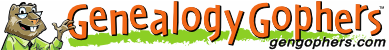# Force in Genealogy Books

Force appears in at least 8423 genealogy books

### Here are the most common given names for Force

Africa Force

America Force

American Force

April Force

Aug Force

August Force

Bill Force

Charles Force

Chief Force

China Force

Christian Force

Church Force

Civil Force

Class Force

Council Force

Court Force

Daniel Force

David Force

Donald Force

Douglas Force

Earl Force

Edward Force

Elizabeth Force

English Force

Field Force

First Force

Forbes Force

Fort Force

Foster Force

France Force

Francis Force

Frank Force

Frederick Force

French Force

Ft Force

Gen Force

George Force

German Force

Henry Force

High Force

Home Force

House Force

Indian Force

Jackson Force

James Force

Jan Force

January Force

Jefferson Force

John Force

Joseph Force

July Force

June Force

Justice Force

King Force

Law Force

Lee Force

Lewis Force

Ll Force

London Force

Lord Force

Louis Force

Lt Force

Maj Force

Major Force

Man Force

March Force

Marine Force

Mary Force

May Force

Men Force

Michael Force

North Force

Park Force

Paul Force

Peace Force

Peter Force

Power Force

Richard Force

Robert Force

Royal Force

Samuel Force

Scott Force

Sea Force

See Force

Sergeant Force

Sir Force

Smith Force

Son Force

South Force

St Force

Staff Force

Sunday Force

Thomas Force

Union Force

Washington Force

Water Force

Wayne Force

West Force

William Force

Ww Force

Didn't find what you are looking for? Search all genealogy books for Force